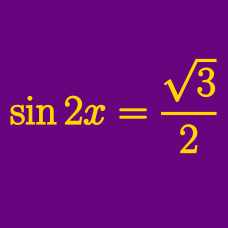Geometry

# Trigonometric Equations - Factoring

In the domain $[0, 2 \pi ]$, how many solutions are there to

$6 \cos^2 \theta - 10 \cos \theta + 3 \sin^2 \theta = 0 ?$

Which of the following are the solutions to the equation

$6 \tan^2 \theta - 17 \tan \theta + 2 \sec^2 \theta = 0 ?$

If $2\pi \leq x < 4\pi$, the sum of all the $x$'s that satisfy $\tan x+ \frac{1}{\tan x}-2=0$ can be expressed as $a\pi$. What is the value of $2a?$

The two roots of the quadratic equation $2x^2-x+k=0$ are $\sin \theta$ and $\cos \theta$. What is $16(\sin^3 \theta+\cos^3 \theta)?$

In the domain $[0^\circ , 1000^\circ ]$, how many solutions are there to $4 \sin^2 \theta - 3 \sin \theta - 1 = 0$?

×